# braindecode.preprocessing.exponential_moving_standardize#

braindecode.preprocessing.exponential_moving_standardize(data, factor_new=0.001, init_block_size=None, eps=0.0001)[source]#

Perform exponential moving standardization.

Compute the exponental moving mean $$m_t$$ at time t as $$m_t=\mathrm{factornew} \cdot mean(x_t) + (1 - \mathrm{factornew}) \cdot m_{t-1}$$.

Then, compute exponential moving variance $$v_t$$ at time t as $$v_t=\mathrm{factornew} \cdot (m_t - x_t)^2 + (1 - \mathrm{factornew}) \cdot v_{t-1}$$.

Finally, standardize the data point $$x_t$$ at time t as: $$x'_t=(x_t - m_t) / max(\sqrt{->v_t}, eps)$$.

Parameters
• data (np.ndarray (n_channels, n_times)) –

• factor_new (float) –

• init_block_size (int) – Standardize data before to this index with regular standardization.

• eps (float) – Stabilizer for division by zero variance.

Returns

standardized – Standardized data.

Return type

np.ndarray (n_channels, n_times)

## Examples using braindecode.preprocessing.exponential_moving_standardize#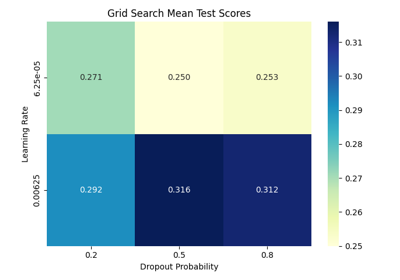Hyperparameter tuning with scikit-learn

Hyperparameter tuning with scikit-learn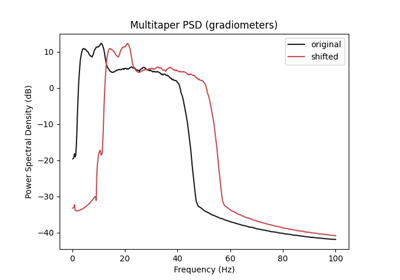Data Augmentation on BCIC IV 2a Dataset

Data Augmentation on BCIC IV 2a Dataset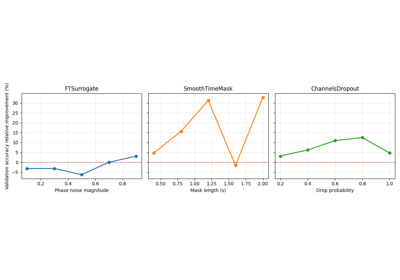Searching the best data augmentation on BCIC IV 2a Dataset

Searching the best data augmentation on BCIC IV 2a Dataset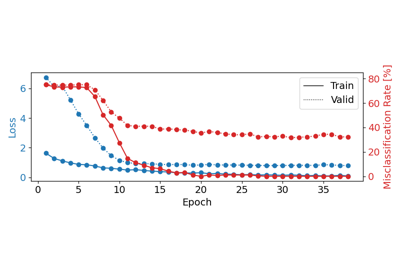Trialwise Decoding on BCIC IV 2a Dataset

Trialwise Decoding on BCIC IV 2a DatasetFingers flexion decoding on BCIC IV 4 ECoG Dataset

Fingers flexion decoding on BCIC IV 4 ECoG DatasetFingers flexion cropped decoding on BCIC IV 4 ECoG Dataset

Fingers flexion cropped decoding on BCIC IV 4 ECoG Dataset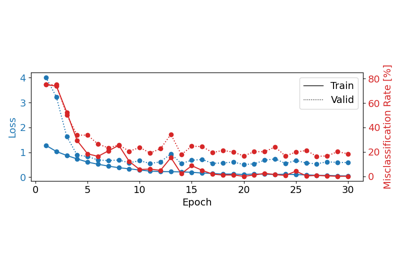Cropped Decoding on BCIC IV 2a Dataset

Cropped Decoding on BCIC IV 2a Dataset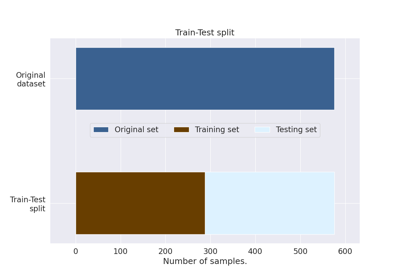How to train, test and tune your model

How to train, test and tune your model